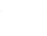#Robo Instructus devblog

Updates on the development of coding puzzler Robo Instructus

## Rust And OpenGL In Orbit Part 3

I'm that guy who quit his job to sit in his house making games. Let's see how this goes! For my previous weekly posts look here.

I’m going to talk a little more about the circle-move-em-up I’ve been going on about these last couple of weeks. When I was developing it, getting circles to draw right then letting you move about, I thought it would be cool to see the curved orbital paths arcing off into the future. But could it be done, and was it actually cool?

### The IdeaThis presented a couple of surmountables

• Calculating where stuff is going to be in the future
• Rendering arbitrary simulated paths

### Seeing into the future

Remember this is a simulation of orbits using gravitational mathematics, so I can’t make too many assumptions about where things are going to go. Actually one assumption I could make is that the user can’t influence the simulation, to I could calculate stuff in advance using that. However, while this is true now this would not be true in an actual game so I’ll ignore it.

I’m going to brute force calculate what happens in the next 50 or so seconds. Currently I’m calculating around 1000 computes a second to adjust the orbit bodies. To properly emulate the master calculation I need to use the same delta in my future projections. So that means 50,000 compute iterations, each giving a future plot point for each body.

The simplest way to implement was just to calculate it each frame. It only makes the compute frames, err 50,000 times slower… Well it’ll probably be fine, how about rendering?

### Drawing the future

Now I’ve computed some plot points I need to draw lines between them. This means figuring out the triangles I want to render on and getting a shader to draw lovely pixels on them. In order to fit in with my alpha-blending anti-aliasing approach used for the bodies I want to draw a line between the plots in some colour and gradually fade the the colour to transparent as we get towards the line thickness.

A line between to points in space can be described by a linear Bézier curve. The shader needs to figure out, based on the pixel position, how close is it to the Bézier line so it can choose an alpha value. It does this by testing points on the line until it can find the closest point, then the alpha (transparency) value will be high if close the line, low if not. Each fragment naturally does this in parallel, shaders are cool!So we just need to do this `50000 * number_of_bodies` times each frame… It turns out this isn’t fine.

### Optimising

At this point I have lines, but by my frames & computes per second have crashed down to ‘not enough’. So even in 2D rendering its easy to kill your frame-rate.

To fix the compute problem I span up a new ‘seer’ thread that will asynchronously calculate the future points and communicate with the main compute thread without blocking it (ie slowing it down). It also continuously calculates points, rather than doing it and discarding every frame. This means the compute thread is no longer affected so much by path calculation, and my CPS sails into the green again. Boom (1/2).

I have too many points to render and actually they’re super close to each other, more than they need to be for the lines to look curved. All I need to do is decide on a good minimum distance and filter out the points which are closer, filtering 50,000 points -> ~1000. Boom (2/2) Actually it’s still slow, even filtering all these points, calculating distance and such, on the CPU is too slow per frame. I move the filtering to the ‘seer’ thread making it responsible for providing renderable points without blocking the main threads. Now the render thread has a manageable amount of points to draw. Boom (2/2).